$$\newcommand{\esp}{\mathbb{E}\left(#1\right)} \newcommand{\var}{\mbox{Var}\left(#1\right)} \newcommand{\deriv}{\dot{#1}(t)} \newcommand{\prob}{ \mathbb{P}\!(#1)} \newcommand{\eqdef}{\mathop{=}\limits^{\mathrm{def}}} \newcommand{\by}{\boldsymbol{y}} \newcommand{\bc}{\boldsymbol{c}} \newcommand{\bpsi}{\boldsymbol{\psi}} \def\pmacro{\texttt{p}} \def\like{{\cal L}} \def\llike{{\cal LL}} \def\logit{{\rm logit}} \def\probit{{\rm probit}} \def\one{{\rm 1\!I}} \def\iid{\mathop{\sim}_{\rm i.i.d.}} \def\simh0{\mathop{\sim}_{H_0}} \def\df{\texttt{df}} \def\res{e} \def\xomega{x} \newcommand{\argmin}{{\rm arg}\min_{#1}} \newcommand{\argmax}{{\rm arg}\max_{#1}} \newcommand{\Rset}{\mbox{\mathbb{R}}} \def\param{\theta} \def\setparam{\Theta} \def\xnew{x_{\rm new}} \def\fnew{f_{\rm new}} \def\ynew{y_{\rm new}} \def\nnew{n_{\rm new}} \def\enew{e_{\rm new}} \def\Xnew{X_{\rm new}} \def\hfnew{\widehat{\fnew}} \def\degree{m} \def\nbeta{d} \newcommand{\limite}{\mathop{\longrightarrow}\limits_{#1}} \def\ka{k{a}} \def\ska{k{\scriptscriptstyle a}} \def\kel{k{e}} \def\skel{k{\scriptscriptstyle e}} \def\cl{C{\small l}} \def\Tlag{T\hspace{-0.1em}{lag}} \def\sTlag{T\hspace{-0.07em}{\scriptscriptstyle lag}} \def\Tk{T\hspace{-0.1em}{k0}} \def\sTk{T\hspace{-0.07em}{\scriptscriptstyle k0}} \def\thalf{t{1/2}} \newcommand{\Dphi}{\partial_\pphi #1} \def\asigma{a} \def\pphi{\psi} \newcommand{\stheta}{{\theta^\star}} \newcommand{\htheta}{{\widehat{\theta}}}$$

# 1 Introduction

A feeding study served to authorize the MON863 maize, a genetically modified organism (GMO) developed by the Monsanto company, by the European and American authorities. It included male and female rats. For each sex, one group was fed with GMOs in the equilibrated diet, and one with the closest control regimen without GMOs.

We are interested in the weight of the rats after a period of 14 weeks.

ratWeight <- read.csv("ratWeight.csv", stringsAsFactors = T)
data <- subset(ratWeight, week==14)
head(data)
##        id week weight  regime gender dosage
## 14 B38602   14  514.9 Control   Male    11%
## 28 B38603   14  505.0 Control   Male    11%
## 42 B38604   14  545.1 Control   Male    11%
## 56 B38605   14  596.6 Control   Male    11%
## 70 B38606   14  516.8 Control   Male    11%
## 84 B38607   14  518.1 Control   Male    11%

The data per gender and regime is displayed below

library(ggplot2)
theme_set(theme_bw())
ggplot(data=data) + geom_point(aes(x=weight,y=as.numeric(regime),colour=regime, shape=gender)) +
ylab(NULL) + scale_y_continuous(breaks=NULL, limits=c(-5,10)) + xlab("weight (g)")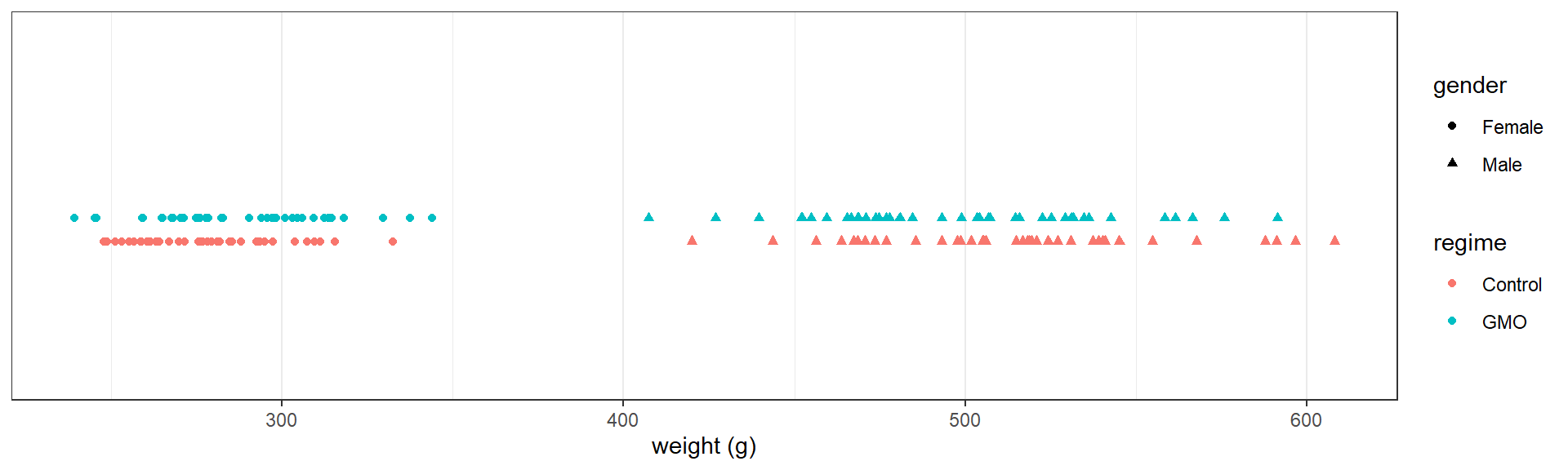The following table provides the mean weight in each group

aggregate(weight ~ regime+gender, data=data, FUN= "mean" )
##    regime gender   weight
## 1 Control Female 278.2825
## 2     GMO Female 287.3225
## 3 Control   Male 513.7077
## 4     GMO   Male 498.7359

Our main objective is to detect some possible effect of the diet on the weight. More precisly, we would like to know if the differences observed in the data are due to random fluctuations in sampling or to differences in diet.

# 2 Student’s t-test

## 2.1 One sample t-test

Before considering the problem of comparing two groups, let us start looking at the weight of the male rats only:

ggplot(data=subset(data,gender=="Male")) + geom_point(aes(x=weight,y=0), colour="red") +
ylab(NULL) + scale_y_continuous(breaks=NULL) + xlab("weight (g)")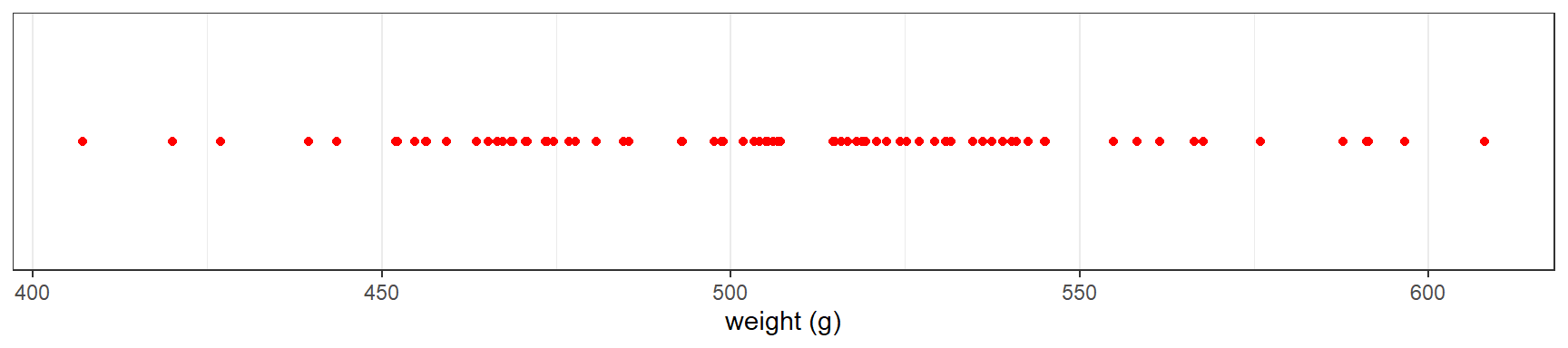Let $$x_1, x_2, x_n$$ the weights of the $$n$$ male rats. We will assume that the $$x_i$$’s are independent and normally distributed with mean $$\mu$$ and variance $$\sigma^2$$:

$x_i \iid {\cal N}(\mu \ , \ \sigma^2)$

### 2.1.1 One sided test

We want to test

$H_0: \ \mu \leq \mu_0" \quad \text{versus} \quad H_1: \ \mu > \mu_0"$

Function t.test can be used for performing this test:

x <- data[datagender=="Male","weight"] mu0 <- 500 t.test(x, alternative="greater", mu=mu0) ## ## One Sample t-test ## ## data: x ## t = 1.2708, df = 77, p-value = 0.1038 ## alternative hypothesis: true mean is greater than 500 ## 95 percent confidence interval: ## 498.0706 Inf ## sample estimates: ## mean of x ## 506.2218 Let us see what these outputs are and how they are computed. Let $$\bar{x} = n^{-1}\sum_{i=1}^n x_i$$ be the empirical mean of the data. $\bar{x} \sim {\cal N}(\mu \ , \ \frac{\sigma^2}{n})$ Then, \begin{aligned} \frac{\sqrt{n}(\bar{x} - \mu)}{\sigma} \ & \sim \ {\cal N}(0 \ , \ 1) \\ \frac{\sqrt{n}(\bar{x} - \mu)}{s} \ & \sim \ t_{n-1} \end{aligned} where $s^2 = \frac{1}{n-1}\sum_{i=1}^n (x_i - \bar{x})^2$ is the empirical variance of the $$x_i$$’s. The statistic used for the test should be a function of the data whose distribution under $$H_0$$ is known, and whose expected behavior under $$H_1$$ allows one to define a rejection region (or critical region) for the null hypothesis. Here, the test statistic is $T_{\rm stat} = \frac{(\bar{x} - \mu_0)}{s/\sqrt{n}}$ which follows a $$t$$-distribution with $$n-1$$ degrees of freedom when $$\mu=\mu_0$$. $$\bar{x}$$ is expected to be less than or equal to $$\mu_0$$ under the null hypothesis, and greater than $$\mu_0$$ under the alternative hypothesis, Hence, $$T_{\rm stat}$$ is expected to be less than or equal to 0 under $$H_0$$ and greater than 0 under $$H_1$$. We then reject the null hypothesis $$H_0$$ if $$T_{\rm stat}$$ is greater than some threshold $$q$$. Such decision rule may lead to two kinds of error: • The type I error is the incorrect rejection of null hypothesis when it is true, • The type II error is the failure to reject the null hypothesis when it is false. The type I error rate or significance level is therefore the probability of rejecting the null hypothesis given that it is true. In our case, for a given significance level $$\alpha$$, we will reject $$H_0$$ if $$T_{\rm stat} > qt_{1-\alpha,n-1}$$, where $$qt_{1-\alpha,n-1}$$ is the quantile of order $$1-\alpha$$ for a $$t$$-distribution with $$n-1$$ degrees of freedom. Indeed, by definition, \begin{aligned} \prob{\text{reject } H_0 \ | \ H_0 \ \text{true}} &= {\mathbb P}(T_{\rm stat} > qt_{1-\alpha,n-1} \ | \ \mu \leq \mu_0) \\ & \leq {\mathbb P}(T_{\rm stat} > qt_{1-\alpha,n-1} \ | \ \mu = \mu_0) \\ & \leq {\mathbb P}(t_{n-1} > qt_{1-\alpha,n-1}) \\ & \leq \alpha \end{aligned} alpha <- 0.05 x.mean <- mean(x) x.sd <- sd(x) n <- length(x) df <- n-1 t.stat <- sqrt(n)*(x.mean-mu0)/x.sd c(t.stat,qt(1-alpha, df)) ##  1.270806 1.664885 We therefore don’t reject $$H_0$$ in our example since $$T_{\rm stat} < qt_{1-\alpha,n-1}$$. We can equivalently compute the significance level for which the test becomes significant. This value is called the p-value: \begin{aligned} p_{\rm value} & = \max{\mathbb P}_{H_0}(T_{\rm stat} > T_{\rm stat}^{\rm obs}) \\ & = {\mathbb P}(T_{\rm stat} > T_{\rm stat}^{\rm obs} \ | \ \mu=\mu_0) \\ &= 1 - \prob{t_{n-1} \leq T_{\rm stat}^{\rm obs}} \end{aligned} Now, $$T_{\rm stat} > qt_{1-\alpha,n-1}$$ under $$H_0$$ if and only if $$\prob{t_{n-1} \leq T_{\rm stat}^{\rm obs}} \geq 1-\alpha$$. Then, the test is significant at the level $$\alpha$$ if and only if $$p_{\rm value}\leq \alpha$$. p.value <- 1 - pt(t.stat,df) print(p.value) ##  0.1038119 Here, we would reject $$H_0$$ for any significance level $$\alpha \geq$$ 0.104. Important: The fact that the test is not significant at the level $$\alpha$$ does not allow us to conclude that $$H_0$$ is true, i.e. that $$\mu$$ is less than or equal to 500. We can only say that the data does not allow us to conclude that $$\mu>500$$. Imagine now that we want to test if $$\mu \geq 515$$ for instance. The alternative here is $$H_1: \ \mu < 515$$. mu0 <- 515 t.test(x, alternative="less", mu=mu0) ## ## One Sample t-test ## ## data: x ## t = -1.793, df = 77, p-value = 0.03845 ## alternative hypothesis: true mean is less than 515 ## 95 percent confidence interval: ## -Inf 514.373 ## sample estimates: ## mean of x ## 506.2218 More generally, we may want to test $H_0: \ \mu \geq \mu_0" \quad \text{versus} \quad H_1: \ \mu < \mu_0"$ We still use the statistic $$T_{\rm stat} = \sqrt{n}(\bar{x}-\mu_0)/s$$ for this test, but the rejection region is now the area that lies to the left of the critical value $$qt_{\alpha,n-1}$$ since \begin{aligned} \prob{\text{reject } H_0 \ | \ H_0 \ \text{true}} &= {\mathbb P}(T_{\rm stat} < qt_{\alpha,n-1} \ | \ \mu \geq \mu_0) \\ & \leq {\mathbb P}(T_{\rm stat} < qt_{\alpha,n-1} \ | \ \mu = \mu_0) \\ & \leq \alpha \end{aligned} t.stat <- sqrt(n)*(x.mean-mu0)/x.sd p.value <- pt(t.stat,df) c(t.stat, df, p.value) ##  -1.79295428 77.00000000 0.03845364 Here, the p-value is less than $$\alpha=0.05$$: we then reject the null hypothesis at the $$5\%$$ level and conclude that $$\mu < 515$$. ### 2.1.2 Two sided test A two sided test (or two tailed test) can be used to test if $$\mu=500$$ for instance mu0 = 500 t.test(x, alternative="two.sided", mu=mu0) ## ## One Sample t-test ## ## data: x ## t = 1.2708, df = 77, p-value = 0.2076 ## alternative hypothesis: true mean is not equal to 500 ## 95 percent confidence interval: ## 496.4727 515.9709 ## sample estimates: ## mean of x ## 506.2218 More generally, we can test $H_0: \ \mu = \mu_0" \quad \text{versus} \quad H_1: \ \mu \neq \mu_0"$ The test also uses the statistic $$T_{\rm stat} = \sqrt{n}(\bar{x}-\mu_0)/s$$, but the rejection region has now two parts: we reject $$H_0$$ if $$|T_{\rm stat}| > qt_{1-\alpha/2}$$. Indeed, \begin{aligned} \prob{\text{reject } H_0 \ | \ H_0 \ \text{true}} &= {\mathbb P}(|T_{\rm stat}| > qt_{1 -\frac{\alpha}{2},n-1} \ | \ \mu = \mu_0) \\ & = {\mathbb P}(T_{\rm stat} < qt_{\frac{\alpha}{2},n-1} \ | \ \mu = \mu_0) + {\mathbb P}(T_{\rm stat} > qt_{1-\frac{\alpha}{2},n-1} \ | \ \mu = \mu_0)\\ &= \prob{t_{n-1} \leq qt_{\frac{\alpha}{2},n-1}} + \prob{t_{n-1} \geq qt_{1-\frac{\alpha}{2},n-1}} \\ &= \frac{\alpha}{2} + \frac{\alpha}{2} \\ & = \alpha \end{aligned} The p-value of the test is now \begin{aligned} p_{\rm value} & = {\mathbb P}_{H_0}(|T_{\rm stat}| > |T_{\rm stat}^{\rm obs}|) \\ & = {\mathbb P}_{H_0}(T_{\rm stat} < -|T_{\rm stat}^{\rm obs}|) + {\mathbb P}_{H_0}(T_{\rm stat} > |T_{\rm stat}^{\rm obs}|)\\ &= \prob{t_{n-1} \leq -|T_{\rm stat}^{\rm obs}|} + \prob{t_{n-1} \geq |T_{\rm stat}^{\rm obs}|} \\ &= 2 \,\prob{t_{n-1} \leq -|T_{\rm stat}^{\rm obs}|} \end{aligned} t.stat <- sqrt(n)*(x.mean-mu0)/x.sd p.value <- 2*pt(-abs(t.stat),df) c(t.stat, df, p.value) ##  1.2708058 77.0000000 0.2076238 Here, $$p_{\rm value}=$$ 0.208. Then, for any significance level less than 0.208, we cannot reject the hypothesis that $$\mu = 500$$. ### 2.1.3 Confidence interval for the mean We have just seen that the data doesn’t allow us to reject the hypothesis that $$\mu = 500$$. But we would come to the same conclusion with other values of $$\mu_0$$. In particular, we will never reject the hypothesis that $$\mu = \bar{x}$$: t.test(x, mu=x.mean, conf.level=1-alpha)p.value
##  1

For a given significance level ($$\alpha = 0.05$$ for instance), we will not reject the null hypothesis for values of $$\mu_0$$ close enough to $$\bar{x}$$.

pv.510 <- t.test(x, mu=510, conf.level=1-alpha)$p.value pv.497 <- t.test(x, mu=497, conf.level=1-alpha)$p.value
c(pv.510, pv.497)
##  0.44265350 0.06340045

On the other hand, we will reject $$H_0$$ for values of $$\mu_0$$ far enough from $$\bar{x}$$:

pv.520 <- t.test(x, mu=520, conf.level=1-alpha)$p.value pv.490 <- t.test(x, mu=490, conf.level=1-alpha)$p.value
c(pv.520, pv.490)
##  0.006204188 0.001406681

There exist two values of $$\mu_0$$ for which the decision is borderline

pv1 <- t.test(x, mu=496.47, conf.level=1-alpha)$p.value pv2 <- t.test(x, mu=515.97, conf.level=1-alpha)$p.value
c(pv1,pv2)
##  0.04993761 0.05001986

In fact, for a given $$\alpha$$, these two values $$\mu_{\alpha,{\rm lower}}$$ and $$\mu_{\alpha,{\rm upper}}$$ define a confidence interval for $$\mu$$: We are confident’’ at the level $$1-\alpha$$ that any value between $$\mu_{\alpha,{\rm lower}}$$ and $$\mu_{\alpha,{\rm upper}}$$ is a possible value for $$\mu$$.

mu <- seq(490,520,by=0.25)
t.stat <- (x.mean-mu)/x.sd*sqrt(n)
pval <- pmin(pt(-t.stat,df) + (1- pt(t.stat,df)),pt(t.stat,df) + (1- pt(-t.stat,df)))
dd <- data.frame(mu=mu, p.value=pval)
CI <- x.mean + x.sd/sqrt(n)*qt(c(alpha/2,1-alpha/2), df)
ggplot(data=dd) + geom_line(aes(x=mu,y=p.value)) +
geom_vline(xintercept=x.mean,colour="red", linetype=2)+
geom_hline(yintercept=alpha,colour="green", linetype=2)+
geom_vline(xintercept=CI,colour="red") +
scale_x_continuous(breaks=round(c(490,500,510,520,CI,x.mean),2))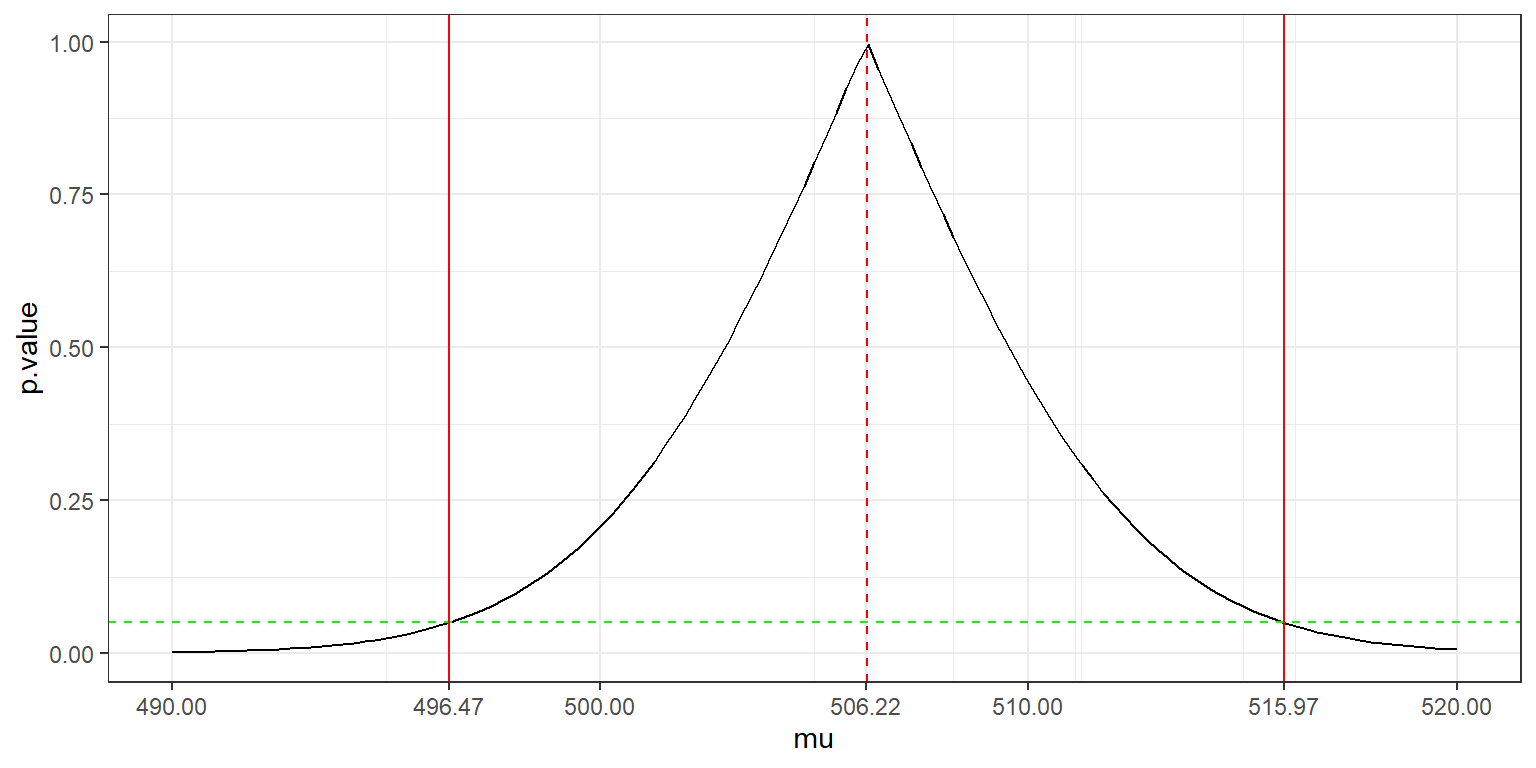By construction,

\begin{aligned} 1-\alpha &= \prob{ qt_{\frac{\alpha}{2},n-1} < \frac{\bar{x}-\mu}{s/\sqrt{n}} < qt_{1-\frac{\alpha}{2},n-1} } \\ &= \prob{ \bar{x} +\frac{s}{\sqrt{n}}qt_{\frac{\alpha}{2},n-1} < \mu < \bar{x} +\frac{s}{\sqrt{n}}qt_{1-\frac{\alpha}{2},n-1} } \end{aligned}

The confidence interval of level $$1-\alpha$$ for $$\mu$$ is therefore the interval ${\rm CI}_{1-\alpha} = [\bar{x} +\frac{s}{\sqrt{n}}qt_{\frac{\alpha}{2},n-1} \ \ , \ \ \bar{x} +\frac{s}{\sqrt{n}}qt_{1-\frac{\alpha}{2},n-1}]$

(CI <- x.mean + x.sd/sqrt(n)*qt(c(alpha/2,1-alpha/2), df))
##  496.4727 515.9709

Remark 1: The fact that $$\prob{ \mu \in {\rm CI}_{1-\alpha}} = 1- \alpha$$ does not mean that $$\mu$$ is a random variable! It is the bounds of the confidence interval that are random because they are function of the data.

A confidence interval of level $$1-\alpha$$ should be interpreted like this: imagine that we repeat the same experiment many times, with the same experimental conditions, and that we build a confidence interval for $$\mu$$ for each of these replicate. Then, the true mean $$\mu$$ will lie in the confidence interval $$(1-\alpha)100\%$$ of the times.

Let us check this property with a Monte Carlo simulation.

L <- 100000
n <- 100
mu <- 500
sd <- 40
R <- vector(length=L)
for (l in (1:L)) {
x <- rnorm(n,mu,sd)
ci.l <- mean(x) + sd(x)/sqrt(n)*qt(c(alpha/2, 1-alpha/2),n-1)
R[l] <- (mu > ci.l  && mu < ci.l)
}
mean(R)
##  0.95043

Remark 2:

The decision rule to reject or not the null hypothesis can be derived from the confidence interval. Indeed, the confidence interval plays the role of an acceptance region: we reject $$H_0$$ if $$\mu_0$$ does not belong to $${\rm CI}_{1-\alpha}$$.

In the case of a one sided test, the output of t.test called confidence interval is indeed an acceptance region for $$\mu$$, but not a confidence interval’’ (we cannot seriouly consider that $$\mu$$ can take any value above 500 for instance )

rbind(
c(x.mean + x.sd/sqrt(n)*qt(alpha,df) , Inf),
c(-Inf, x.mean + x.sd/sqrt(n)*qt(1-alpha,df)))
##          [,1]     [,2]
## [1,] 499.0229      Inf
## [2,]     -Inf 513.4207

## 2.2 Two samples t-test

### 2.2.1 What should we test?

Let us now compare the weights of the male and female rats.

y <- data[data$gender=="Female" ,"weight"] dmean <- data.frame(x=c(mean(x),mean(y)),gender=c("Male","Female")) ggplot(data=subset(data,regime=="Control")) + geom_point(aes(x=weight,y=0,colour=gender)) + geom_point(data=dmean, aes(x,y=0,colour=gender), size=4) + ylab(NULL) + scale_y_continuous(breaks=NULL) + xlab("weight (g)")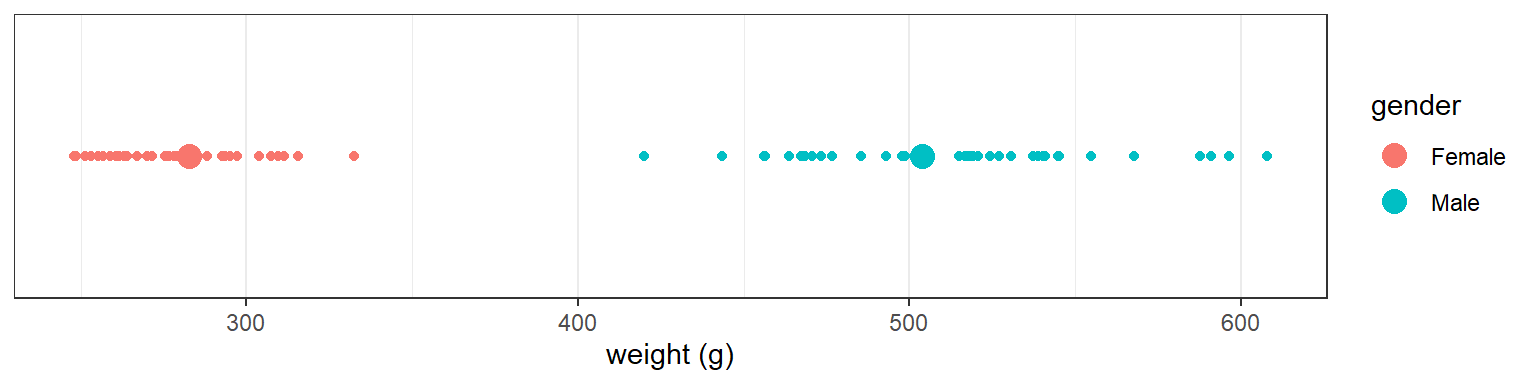Looking at the data is more than enough for concluding that the mean weight of the males is (much) larger than the mean weight of the females Computing a $$p$$-value here is of little interest t.test(x, y) ## ## Welch Two Sample t-test ## ## data: x and y ## t = 49.442, df = 169.85, p-value < 2.2e-16 ## alternative hypothesis: true difference in means is not equal to 0 ## 95 percent confidence interval: ## 212.1025 229.7438 ## sample estimates: ## mean of x mean of y ## 503.7257 282.8025 Let us see now what happens if we compare the control and GMO groups for the male rats. x <- data[data$gender=="Male" & data$regime=="Control","weight"] y <- data[data$gender=="Male" & data$regime=="GMO","weight"] dmean <- data.frame(x=c(mean(x),mean(y)),regime=c("Control","GMO")) ggplot(data=data[data$gender=="Male",]) +  geom_point(aes(x=weight,y=as.numeric(regime),colour=regime)) +
geom_point(data=dmean, aes(x,y=as.numeric(regime),colour=regime), size=4) +
ylab(NULL) + scale_y_continuous(breaks=NULL, limits=c(-6,9)) + xlab("weight (g)")
## Warning in FUN(X[[i]], ...): NAs introduits lors de la
## conversion automatique
## Warning: Removed 2 rows containing missing values
## (geom_point).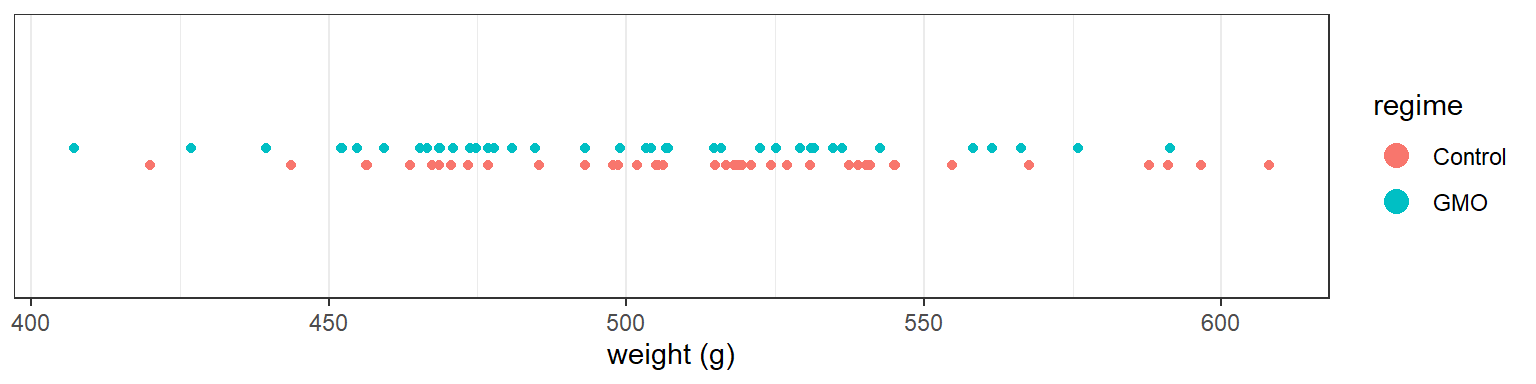We observe a difference between the two empirical means (the mean weight after 14 weeks is greater in the control group), but we cannot say how significant this difference is by simply looking at the data. Performing a statistical test is now necessary.

Let $$x_{1}, x_{2}, \ldots, x_{n_x}$$ be the weights of the $$n_x$$ male rats of the control group and $$y_{1}, y_{2}, \ldots, y_{n_x}$$ the weights of the $$n_y$$ male rats of the GMO group. We will assume normal distributions for both $$(x_{i})$$ and $$(y_{i})$$:

$x_{i} \iid {\cal N}(\mu_x \ , \ \sigma^2_x) \quad ; \quad y_{i} \iid {\cal N}(\mu_y \ , \ \sigma^2_y)$

We want to test

$H_0: \ \mu_x = \mu_y" \quad \text{versus} \quad H_1: \ \mu_x \neq \mu_y"$

### 2.2.2 Assuming equal variances

We can use the function t.test assuming first equal variances ($$\sigma^2_x=\sigma_y^2$$)

alpha <- 0.05
t.test(x, y, conf.level=1-alpha, var.equal=TRUE)
##
##  Two Sample t-test
##
## data:  x and y
## t = 1.5426, df = 76, p-value = 0.1271
## alternative hypothesis: true difference in means is not equal to 0
## 95 percent confidence interval:
##  -4.358031 34.301621
## sample estimates:
## mean of x mean of y
##  513.7077  498.7359

The test statistic is $T_{\rm stat} = \frac{\bar{x} - \bar{y}}{s_p \sqrt{\frac{1}{n_x}+\frac{1}{n_y}}}$ where $$s_p^2$$ is the pooled variance:

$s_p^2 = \frac{1}{n_x+n_y-2} \left(\sum_{i=1}^{n_x} (x_{i}-\bar{x})^2 + \sum_{i=1}^{n_y} (y_{i}-\bar{y})^2 \right)$

Under the null hypothesis, $$T_{\rm stat}$$ follows a $$t$$-distribution with $$n_x+n_y-2$$ degree of freedom. The $$p$$-value is therefore

\begin{aligned} p_{\rm value} & = {\mathbb P}_{H_0}(|T_{\rm stat}| > |T_{\rm stat}^{\rm obs}|) \\ &= \prob{t_{n_x+n_y-2} \leq -T_{\rm stat}^{\rm obs}} + 1 - \prob{t_{n_x+n_y-2} \leq T_{\rm stat}^{\rm obs}} \end{aligned}

nx <- length(x)
ny <- length(y)
x.mean <- mean(x)
y.mean <- mean(y)
x.sc <- sum((x-x.mean)^2)
y.sc <- sum((y-y.mean)^2)
xy.sd <- sqrt((x.sc+y.sc)/(nx+ny-2))
t.stat <- (x.mean-y.mean)/xy.sd/sqrt(1/nx+1/ny)
df <- nx + ny -2
p.value <- pt(-t.stat,df) + (1- pt(t.stat,df))
c(t.stat, df, p.value)
##   1.5426375 76.0000000  0.1270726

The confidence interval for the mean difference $$\mu_x-\mu_y$$ is computed as ${\rm CI}_{1-\alpha} = [\bar{x} - \bar{y} +s_p \sqrt{\frac{1}{n_x}+\frac{1}{n_y}}qt_{\frac{\alpha}{2},n_x+n_y-2} \ \ , \ \ \bar{x} - \bar{y} +s_p \sqrt{\frac{1}{n_x}+\frac{1}{n_y}}qt_{1-\frac{\alpha}{2},n_x+n_y-2} ]$

x.mean-y.mean  + xy.sd*sqrt(1/nx+1/ny)*qt(c(alpha/2,1-alpha/2),df)
##  -4.358031 34.301621

### 2.2.3 Assuming different variances

Assuming equal variances for the two groups may be disputable.

aggregate(data$weight ~ data$regime, FUN= "sd" )
##   data$regime data$weight
## 1     Control    123.0689
## 2         GMO    111.8559

We can then use the t.test function with different variances (which is the default)

t.test(x, y, conf.level=1-alpha)
##
##  Welch Two Sample t-test
##
## data:  x and y
## t = 1.5426, df = 75.976, p-value = 0.1271
## alternative hypothesis: true difference in means is not equal to 0
## 95 percent confidence interval:
##  -4.358129 34.301719
## sample estimates:
## mean of x mean of y
##  513.7077  498.7359

The Welch (or Satterthwaite) approximation to the degrees of freedom is used instead of $$n_x+n_y-2=$$ 76:

$\df_W = \frac{(c_x + c_y)^2}{{c_x^2}/{(n_x-1)} + {c_y^2}/{(n_y-1)}}$ where $$c_x = \sum (x_{i}-\bar{x})^2/(n_x(n_x-1))$$ and $$c_y = \sum (y_{i}-\bar{y})^2/(n_y(n_y-1))$$.

Furthermore, unlike in Student’s t-test with equal variances, the denominator is not based on a pooled variance estimate:

$T_{\rm stat} = \frac{\bar{x} - \bar{y}}{ \sqrt{{s_x^2}/{n_x}+{s_y^2}/{n_y}}}$ where $$s_x^2$$ and $$s_y^2$$ are the empirical variances of $$(x_i)$$ and $$(y_i)$$: $s_x^2 = \frac{1}{n_x-1}\sum_{i=1}^{n_x} (x_{i}-\bar{x})^2 \quad ; \quad s_y^2 = \frac{1}{n_y-1}\sum_{i=1}^{n_y} (y_{i}-\bar{y})^2$

sbar.xy <- sqrt(var(x)/nx+var(y)/ny)
t.stat <- (x.mean-y.mean)/sbar.xy
cx <- x.sc/(nx-1)/nx
cy <- y.sc/(ny-1)/ny
dfw <- (cx + cy)^2 / (cx^2/(nx-1) + cy^2/(ny-1))
p.value <- pt(-t.stat,dfw) + (1- pt(t.stat,dfw))
c(t.stat, dfw, p.value)
##   1.5426375 75.9760868  0.1270739

The confidence interval for $$\mu_x-\mu_y$$ is now computed as ${\rm CI}_{1-\alpha} = [\bar{x} - \bar{y} +\sqrt{\frac{s_x^2}{n_x}+\frac{s_y^2}{n_y}} \ qt_{\frac{\alpha}{2},\df_W} \ \ , \ \ \bar{x} - \bar{y} +\sqrt{\frac{s_x^2}{n_x}+\frac{s_y^2}{n_y}} \ qt_{1-\frac{\alpha}{2},\df_W} ]$

x.mean-y.mean  + sbar.xy*qt(c(alpha/2,1-alpha/2),dfw)
##  -4.358129 34.301719

## 2.3 Power of a t-test

Until now, we have demonstrated that the experimental data does not highlight any significant difference in weight between the control group and the GMO group.

Of course, that does not mean that there is no difference between the two groups. Indeed, absence of evidence is not evidence of absence. In fact, no experimental study would be able to demonstrate the absence of effect of the diet on the weight.

Now, the appropriate question is rather to evaluate what the experimental study can detect. If feeding a population of rats with GMOs has a signicant biological effect on the weight, can we ensure with a reasonable level of confidence that our statistical test will reject the null hypothesis and conclude that there is indeed a difference in weight between the two groups?

A power analysis allows us to determine the sample size required to detect an effect of a given size with a given degree of confidence. Conversely, it allows us to determine the probability of detecting an effect of a given size with a given level of confidence, under sample size constraints.

For a given $$\delta \in {\mathbb R}$$, let $$\beta(\delta)$$ be the type II error rate, i.e. the probability to fail rejecting $$H_0$$ when $$\mu_x-\mu_y = \delta$$, with $$\delta\neq 0$$.

The power of the test is the probability to reject the null hypothesis when it is false. It is also a function of $$\delta =\mu_x-\mu_y$$ defined as

\begin{aligned} \eta(\delta) &= 1 - \beta(\delta) \\ &= \prob{\text{reject } H_0 \ | \ \mu_x-\mu_y=\delta } \end{aligned}

Remember that, for a two sided test, we reject the null hypothesis when $$|T_{\rm stat}| > qt_{1-\alpha/2, \df}$$, where $$\df$$ is the appropriate degree of freedom.

On the other hand, $${(\bar{x} - \bar{y} - \delta)}/{s_{xy}}$$, where $$s_{xy} = \sqrt{{s_x^2}/{n_x}+{s_y^2}/{n_y}}$$, follows a $$t$$-distribution with $$\df$$ degrees of freedom. Thus,

\begin{aligned} \eta(\delta) &= 1 - {\mathbb P}(qt_{\frac{\alpha}{2},\df} < T_{\rm stat} < qt_{1 -\frac{\alpha}{2},\df} \ | \ \mu_x-\mu_y=\delta) \\ & = 1- {\mathbb P}(qt_{\frac{\alpha}{2},\df} < \frac{\bar{x} - \bar{y}}{s_{xy}} < qt_{1-\frac{\alpha}{2},\df} \ | \ \mu_x-\mu_y=\delta) \\ &= 1- {\mathbb P}(qt_{\frac{\alpha}{2},\df} - \frac{\delta}{s_{xy}} < \frac{\bar{x} - \bar{y} - \delta}{s_{xy}} < qt_{1-\frac{\alpha}{2},\df} - \frac{\delta}{s_{xy}} \ | \ \mu_x-\mu_y=\delta) \\ &= 1 - Ft_{\df}(qt_{1-\frac{\alpha}{2},\df} - \frac{\delta}{s_{xy}}) + Ft_{\df}(qt_{\frac{\alpha}{2},\df} - \frac{\delta}{s_{xy}}) \end{aligned}

As an example, let us compute the probability to detect a difference in weight of 10g with two groups of 80 rats each and assuming that the standard deviation is 30g in each group.

alpha=0.05
nx.new <- ny.new <- 80
delta.mu <- 10
x.sd <- 30
df <- nx.new+ny.new-2
dt <- delta.mu/x.sd/sqrt(1/nx.new+1/ny.new)
1-pt(qt(1-alpha/2,df)-dt,df) + pt(qt(alpha/2,df)-dt,df) 
##  0.5528906

The function pwr.t.test allows to compute this power:

library(pwr)
pwr.t.test(n=nx.new, d=delta.mu/x.sd, type="two.sample", alternative="two.sided", sig.level=alpha)
##
##      Two-sample t test power calculation
##
##               n = 80
##               d = 0.3333333
##       sig.level = 0.05
##           power = 0.5538758
##     alternative = two.sided
##
## NOTE: n is number in *each* group

Let us perform a Monte Carlo simulation, to check this result and better understand what it means. Imagine that the true’’ difference in weight is $$\delta=10$$g. The